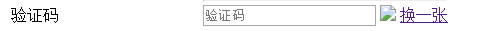JS图片验证码换一张不显示name="checkcode" placeholder="验证码">

class="verifyimg"
src="\${pageContext.request.contextPath}/UserServlet?method=CheckCode" />

``````            function changeImg() {
var img = document.getElementById("imgId");
img.src = "\${pageContext.request.contextPath}/UserServlet?method=CheckCode?" + new Date();
}

public void CheckCode(HttpServletRequest req, HttpServletResponse resp) throws IOException {

// 使用java图形界面技术绘制一张图片

int charNum = 4;
int width = 30 * 4;
int height = 30;

// 1. 创建一张内存图片
BufferedImage bufferedImage = new BufferedImage(width, height, BufferedImage.TYPE_INT_RGB);

// 2.获得绘图对象
Graphics graphics = bufferedImage.getGraphics();

// 3、绘制背景颜色
graphics.setColor(Color.YELLOW);
graphics.fillRect(0, 0, width, height);

// 4、绘制图片边框
graphics.setColor(Color.BLUE);
graphics.drawRect(0, 0, width - 1, height - 1);

// 5、输出验证码内容
graphics.setColor(Color.RED);
graphics.setFont(new Font("宋体", Font.BOLD, 20));

// 随机输出4个字符
Graphics2D graphics2d = (Graphics2D) graphics;
String s = "ABCDEFGHGKLMNPQRSTUVWXYZ23456789";
Random random = new Random();
// session中要用到
String msg = "";
int x = 5;
for (int i = 0; i < 4; i++) {
int index = random.nextInt(32);
String content = String.valueOf(s.charAt(index));
msg += content;
double theta = random.nextInt(45) * Math.PI / 180;
// 让字体扭曲
graphics2d.rotate(theta, x, 18);
graphics2d.drawString(content, x, 18);
graphics2d.rotate(-theta, x, 18);
x += 30;
}

// 6、绘制干扰线
graphics.setColor(Color.GRAY);
for (int i = 0; i < 5; i++) {
int x1 = random.nextInt(width);
int x2 = random.nextInt(width);

int y1 = random.nextInt(height);
int y2 = random.nextInt(height);
graphics.drawLine(x1, y1, x2, y2);
}

// 释放资源
graphics.dispose();

// 图片输出 ImageIO
ImageIO.write(bufferedImage, "jpg", resp.getOutputStream());

}
``````

3个回答a19892213 单独写个验证码servlet就解决了1进击的小白 回复a19892213: 解决了没？是哪的问题1进击的小白 回复a19892213: 看你后台返回的数据，我这边的是byte[] bytes = ValidateHelper.CreateValidateGraphic(out code, 4, 100, 37, 20); return File(bytes, @"image/jpeg");a19892213 method: CheckCode? d=0.9965755928756856， 但是点击换一张还是显示不出来